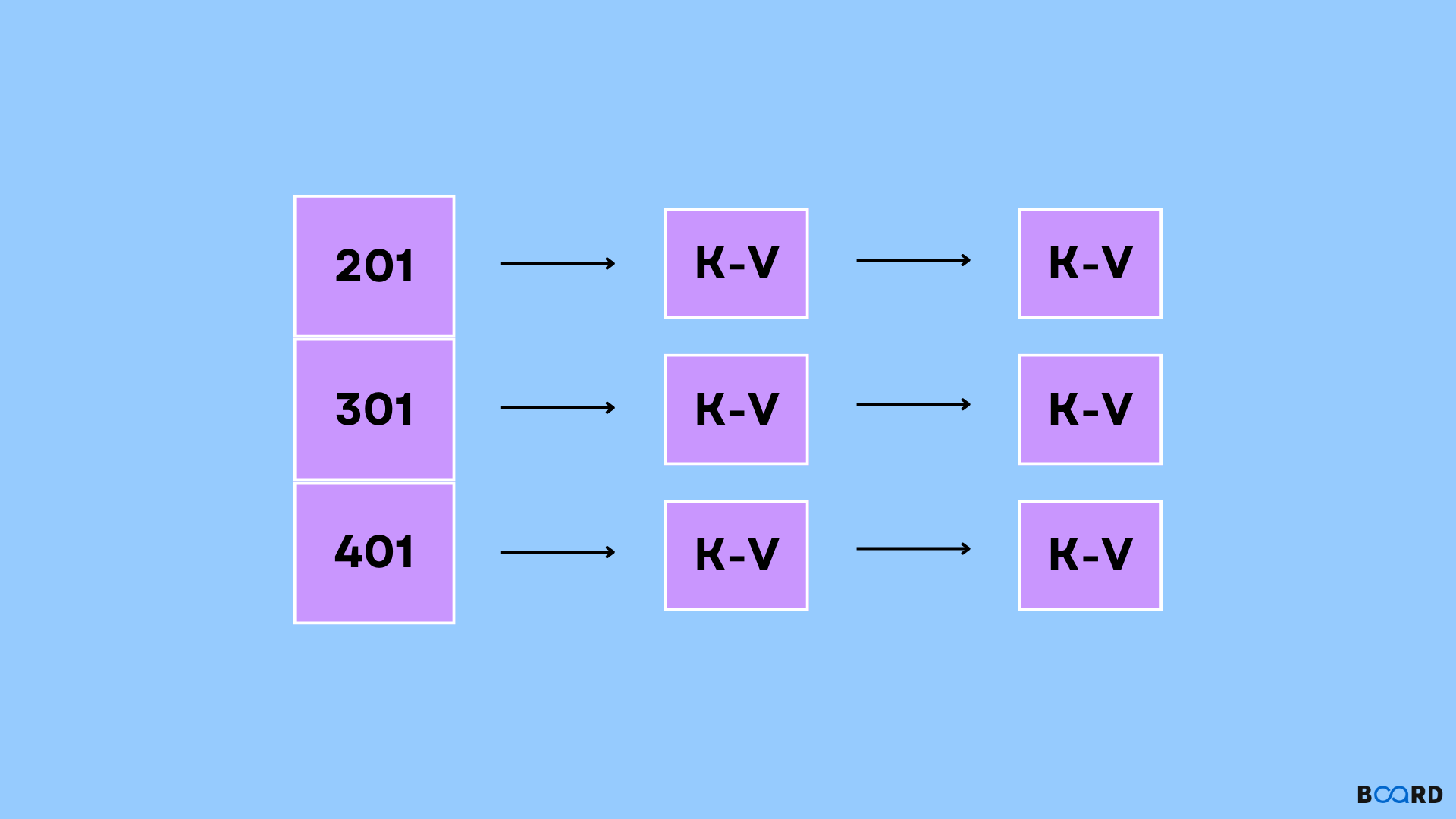## Overview

Hash-Map is an important data structure we use to store pairs of keys and values. Operations such as insert, search, etc work in a constant time complexity. Rehashing is an essential concept in a hashmap. Before we understand rehashing, we must learn about load factoring. The load factor is a measure of how full the hash table can get before its capacity must be increased. The load factor is a metric of the performance of the hash map.

## Basics of Hashing

Before continuing with an in-depth analysis of rehashing and load factoring, let us have a quick look at the basics of hashing:

• The first hash code of key ‘K’ is computed, using the internal hash algorithm, such as murmur3, xxxhash, etc.
• This hash code is used in determining the index position of the bucket, which is an array and stores the nodes on the inside.
• The object is inserted If it does not already exist at that index. Or else, its value is updated as a node in the list.

The value of K, along with the hash function, would determine the time that will be required to finish the first step. If the k is a string, the hash method will be dependent upon its size. But for big values of N, the count of map entries, and the size of the keys is virtually small in comparison to N. Thus we infer that the time complexity of the hash computation is constant.

Now comes the part where we traverse the key-value pairs list at that index. The worst-case would be that each of the N entries is present at the same position, making the time complexity O(N). But hashing algorithms ensure that the hash-maps are sufficiently distributed, so this condition barely occurs. For entries and B array size, every index has N/B entries, which is the load factor.

## What is Rehashing?

The phenomenon of recomputing the hash code of previously-stored key-value pairs, so as to replace them to a bigger size hashmap once the threshold is crossed. Rehashing is done every time the count of elements in a hash map approaches the maximum value of the threshold.

## Why is rehashing needed?

Rehashing is required so that anytime a new entry is stored on the map the load factor increases and so does time complexity. Therefore we use rehashing to distribute the entries across the hashmap. This way we lower both, the time complexity as well as the load factor.

## How is Rehashing Done?

• Add a new entry to the map and compute the load factor.
• If the load factor is greater than the pre-defined/default value, rehash.
• Create a new array of buckets twice the size of the previous one.
• For every element in the old bucket array call the insert function to add it to the bigger and newer bucket array.